# Recognition and Elimination of Subharmonic OscillationsGet valuable resources straight to your inbox - sent out once per month

## Introduction

DC/DC instability occurs due to a variety of factors, such as improper compensation parameters or insufficient layout. This article will discuss subharmonic oscillations, a form of instability that can be generated when current mode switching regulators have a continuous inductor current and a duty cycle exceeding 50%. These oscillations then create unstable power supplies.

To learn about subharmonic oscillations, consider a buck circuit with peak current control, and how these oscillations may change based in relation to time and frequency.

## Subharmonic Oscillations in Relation to Time

Figure 1 shows a buck circuit with peak current control that compares the error signal (VC) with the inductor current signal (IL). VC is obtained by subtracting the reference voltage (VREF) from the output voltage (VOUT). This generates a control signal to drive the upper and lower MOSFETs.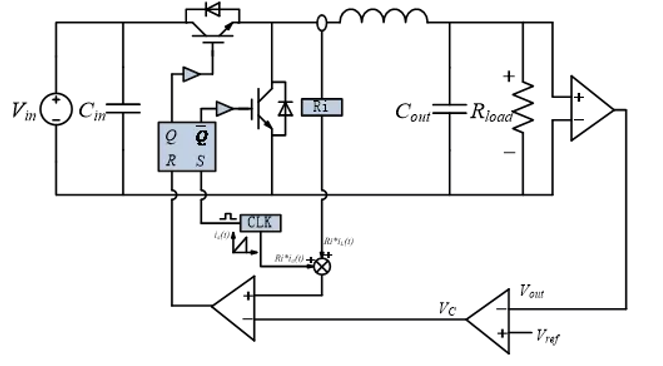Figure 1: Comparison of VC and IL

Figure 2 shows where VC and IL intersect. The blue line represents the IL signal and the switching waveform (SW) under stable conditions, while the pink line represents the IL signal and SW waveform after disturbances. ∆I0 and ∆tON are defined by the SW as the error value. Figure 2 shows that at a duty ratio of 30%, the disturbance gradually decreases, and the system tends to be stable.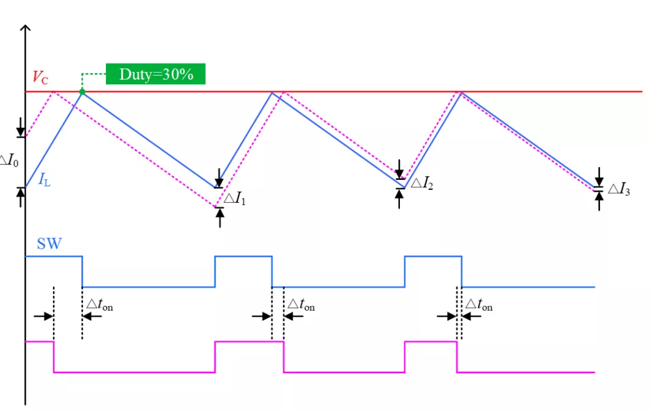Figure 2: Relatively Stable IL at Duty Cycle = 30%

Figure 3 shows that when the duty ratio increases to 70%, the disturbance gradually increases, resulting in system instability.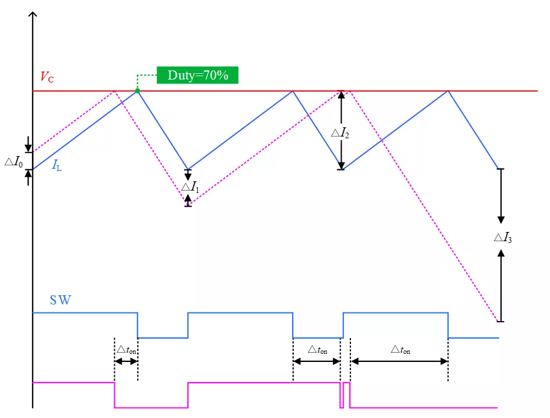Figure 3: Unstable IL at Duty Cycle = 70%

Figure 2 and Figure 3 show that a duty ratio of 50% can be inferred as the boundary point between the convergence and divergence of the disturbance.

The boundary point can also be formulaically derived. Figure 4 shows the inductor current disturbance waveform. The variables are defined where D represents the duty cycle, m1 and m2 are the inductor current’s rising and falling slopes, and ic1 and ic2 are the value when the inductor current reaches VC. ∆iL(0) and ∆iLT(s) on the pink dotted line represent the inductor current’s beginning and end values, respectively. iL(0) and iLT(s) on the blue solid line represent the disturbance’s beginning and end values, respectively.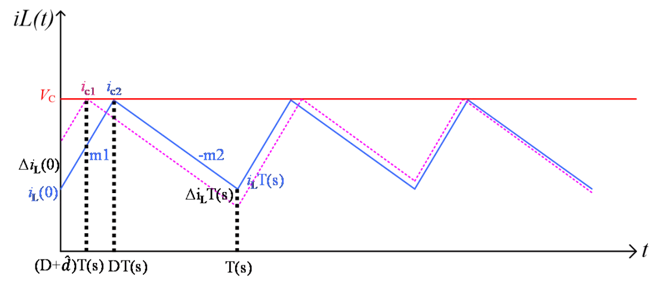Figure 4: Inductor Current Disturbance Waveform

Using these defined variables, ΔiLT can be calculated using Equation (1):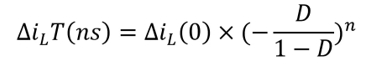For example, when D is less than half (meaning the duty cycle is below 50%) in the nth cycle, ΔiLT gradually converges to zero. Conversely, if the duty cycle exceeds 50%, ∆iLT diverges. The disturbance is determined to be a subharmonic oscillation because the value can be positive or negative, is n-related, and has a rate of change that is exactly half of the switching frequency.

## Subharmonic Oscillations in Relation to Frequency

We can also obtain the next subharmonic oscillation using the frequency domain. Based on the buck circuit diagram with peak current control from Figure 1, Figure 5 shows a system structure diagram that can derive the open-loop transfer function, which is controlled by the system to the output.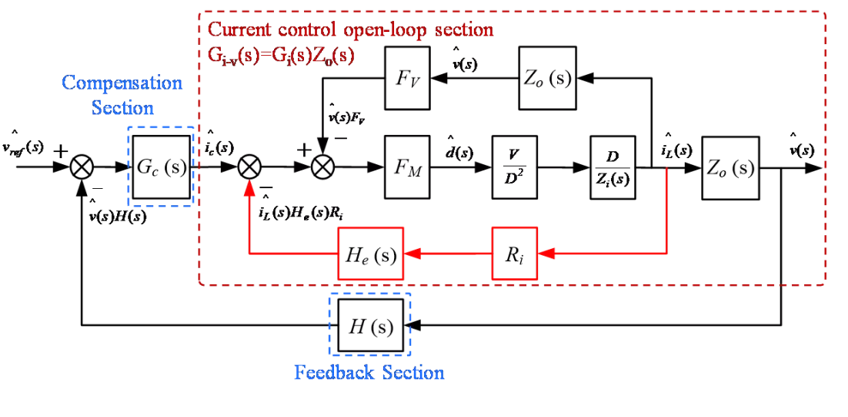Figure 5: Open-Loop Transfer in Buck Circuit System with Peak Current Control

With the open-loop transfer function controlled to the output, the input voltage (VIN) is set to 12V, and the switching frequency (fSW) is 400kHz, the duty cycle changes in accordance with the Bode diagram. Figure 6 shows that when the duty is at 50% and 67%, the gain curve has a resonance peak at half of the switching frequency. The phase curve flips quickly, indicating that subharmonic oscillation has occurred. This significantly affects system stability. However, when the duty cycle is at 28%, neither the gain curve nor the phase curve is abnormal.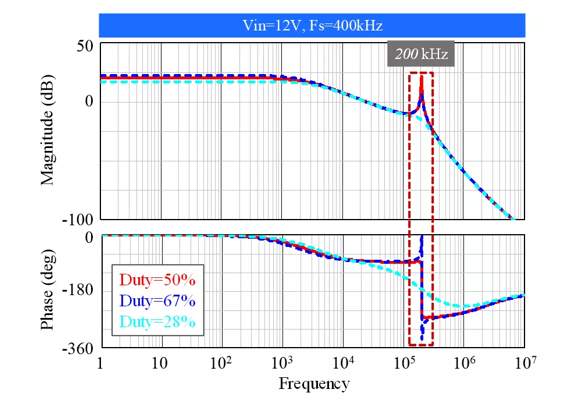Figure 6: Gi-v(s) Function Bode Diagram

## Eliminating Subharmonic Oscillations

There are several methods to reduce subharmonic oscillations. Figure 7 shows the peak current control block diagram with sawtooth wave current compensation. For this method, a sawtooth wave compensation signal is superimposed on the inductor current signal. As a result, the VC signal changes from a constant value to a pulsating slope signal. Typically, this process is referred to as the slope compensation technique.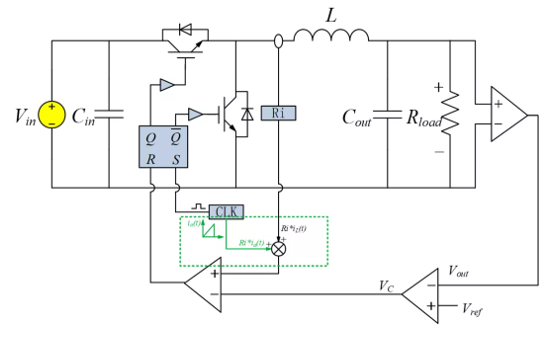Figure 7: Incorporation of Sawtooth Wave Current Compensation

Figure 8 shows the suppression effect of the slope compensation technique by comparing what happens when there is slope compensation to when there is not.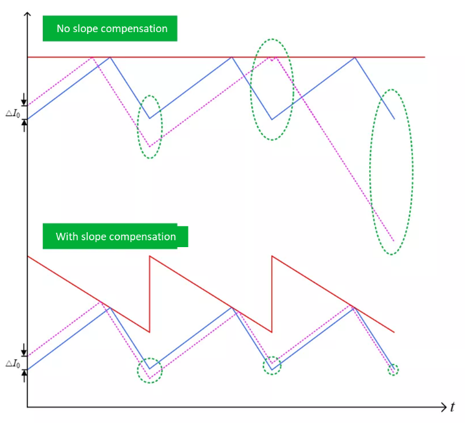Figure 8: Suppression Effect with Sawtooth Wave Current Compensation

The slope compensation technique can be analyzed using mathematical derivations and different frequencies.

## Drawbacks of the Slope Compensation Technique

Although slope compensation can suppress subharmonic oscillations, consider the drawbacks of using of slope compensation in the power supply design process, described below:

1. Due to the addition of slope compensation, the chip’s current limit gradually decreases with an increasing duty cycle.
2. Excessive slope compensation affects the system’s dynamic performance.

Consider the MPQ4420A, a high-efficiency, synchronous step-down converter with built-in power MOSFETs. Figure 9 shows that a larger duty cycle corresponds with a smaller current limit point.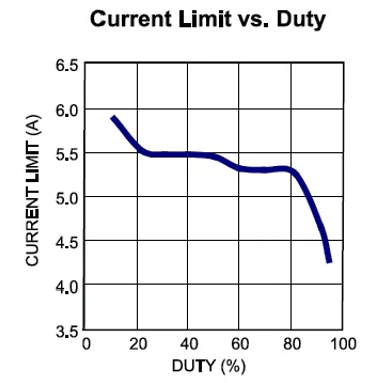Figure 9: The MPQ4420A’s Current Limit vs. Duty Cycle

Figure 10 shows the Gi-v(s) function with slope compensation, where Mc is the slope compensation coefficient. The larger the compensation coefficient, the smaller the system’s bandwidth, and the slower the system's dynamic response.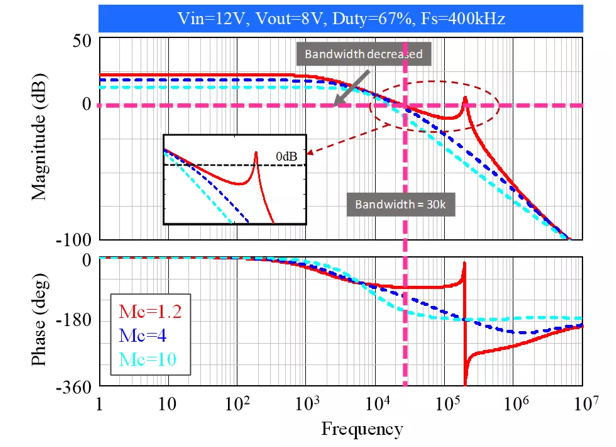Figure 10: Gi-v(s) Bode Diagram with Slope Compensation

## Conclusion

In conclusion, it is crucial to control subharmonic oscillations to maintain switching power supply stability. In this article, we discussed how to recognize subharmonic oscillations using the time domain and frequency domains. We also covered how slope compensation can reduce subharmonic oscillations despite its negative effects.

MPS provides leading power solutions for DC/DC stability, including our Isolated DC/DC Converters & Modules.

### Did you find this interesting? Get valuable resources straight to your inbox - sent out once per month!

Get technical support